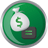Simple InterestQuantitative Aptitude Questions and Answers section on “Simple Interest” with solution and explanation for competitive examinations such as CAT, MBA, SSC, Bank PO, Bank Clerical and other examinations.

The simple interest on 7300 Rs. from 11 May, 1987 to 10 september, 1987 (both days included) at 5% per annum is :

The simple interest on 7300 Rs. from 11 May, 1987 to 10 september, 1987 (both days included) at 5% per annum is : [A]103 Rs. [B]123 Rs. [C]200 Rs. [D]223 Rs. Show Answer 123 Rs. Time from 11 May to 10 September, 1987 = 21 + 30 + 31 + 31 + 10 = 123 ..

A man had 16000 Rs., part of which he lent at 4% and the rest at 5% per annum simple interest. If the total interest received was 700 Rs. In one year, the money lent at 4% per annum was :

A man had 16000 Rs., part of which he lent at 4% and the rest at 5% per annum simple interest. If the total interest received was 700 Rs. In one year, the money lent at 4% per annum was : [A]6000 Rs. [B]8000 Rs. [C]10000 Rs. [D]12000 Rs. Show Answer 10000 Rs. Let the ..

A man lent 60000 Rs. partly at 5% and the rest at 4% simple interest. If the total annual interest is 2560 Rs. the money lent at 4% was :

A man lent 60000 Rs. partly at 5% and the rest at 4% simple interest. If the total annual interest is 2560 Rs. the money lent at 4% was : [A]30000 [B]40000 [C]44000 [D]45000 Show Answer 44000 Let the amount lent at 4% be x ∴ Amount lent at 5% = (60000 – x) According ..